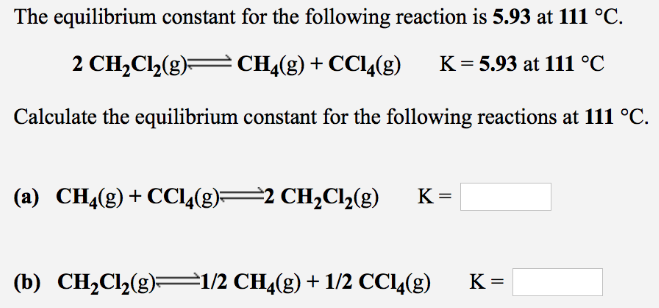# Problem: The equilibrium constant for the following reaction is 5.93 at 111°C. 2CH2Cl2 (g) ⇌ CH4 (g) + CCl4 (g)       K = 5.93 at 111°CCalculate the equilibrium constant for the following reactions at 111 °C. (a) CH4 (g) + CCl4 (g) ⇌ 2CH2Cl2 (g)      K = ?(b) CH2Cl2 (g) ⇌ 1/2CH4 (g) + 1/2CCl4 (g)    K = ?

###### FREE Expert Solution
96% (310 ratings)###### Problem Details

The equilibrium constant for the following reaction is 5.93 at 111°C.

2CH2Cl2 (g) ⇌ CH4 (g) + CCl4 (g)       K = 5.93 at 111°C

Calculate the equilibrium constant for the following reactions at 111 °C.

(a) CH4 (g) + CCl4 (g) ⇌ 2CH2Cl2 (g)      K = ?

(b) CH2Cl2 (g) ⇌ 1/2CH4 (g) + 1/2CCl4 (g)    K = ?